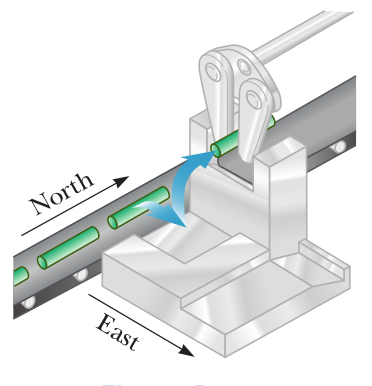Physics Practice Problems Vector Addition & Subtraction Practice Problems Solution: In an assembly operation illustrated in the figure...

🤓 Based on our data, we think this question is relevant for Professor Schulte's class at UCF.

# Solution: In an assembly operation illustrated in the figure, a robot moves an object first straight upward and then also to the east around an arc forming one-quarter of a circle of radius 4.80 cm that lies in an east-west vertical plane. The robot then moves the object upward and to the north through one-quarter of a circle of radius 3.70 cm that lies in a north- south vertical plane. Find (a) the magnitude of the total displacement of the object and (b) the angle the total displacement makes with the vertical.￼

###### Problem

In an assembly operation illustrated in the figure, a robot moves an object first straight upward and then also to the east around an arc forming one-quarter of a circle of radius 4.80 cm that lies in an east-west vertical plane. The robot then moves the object upward and to the north through one-quarter of a circle of radius 3.70 cm that lies in a north- south vertical plane. Find (a) the magnitude of the total displacement of the object and (b) the angle the total displacement makes with the vertical.###### Solution

We're looking for the resultant displacement of an object moved in two separate steps by an assembly machine.

When adding vectors in 3D, the steps are basically the same as adding vectors in 2D, except that drawing diagrams for 3D problems is often skipped because it's more complicated to do.

1. Resolve the vectors into components, if necessary.
3. Add or subtract vectors as required.
4. Convert back to magnitude-angle notation (unless the problem only asks for components).

For step 1, we'll usually use equations of this form to find our components when the components aren't given to us:

,  ,

For step 4, we'll use the same equations from step 1 solved for α, plus the equation for magnitude:

$\overline{)\mathbf{|}\stackrel{\mathbf{⇀}}{\mathbit{A}}\mathbf{|}{\mathbf{=}}\sqrt{{{\mathbit{A}}_{\mathbit{x}}}^{\mathbf{2}}\mathbf{+}{{\mathbit{A}}_{\mathbit{y}}}^{\mathbf{2}}\mathbf{+}{{\mathbit{A}}_{\mathbit{z}}}^{\mathbf{2}}}}$

This problem looks tricky at first because it's very wordy! But let's tackle it one piece at a time.View Complete Written Solution#### Q. Vector A S has a magnitude of 29 units and points in the positive y direction. When vector B S is added to A S the resultant vector A S 1 B S points i...

Solved • Mon Oct 29 2018 16:36:12 GMT-0400 (EDT)

#### Q. Vector A S has x and y components of 28.70 cm and 15.0 cm respectively vector B S has x and y components of 13.2 cm and 26.60 cm respectively. If A S ...

Solved • Mon Oct 29 2018 16:36:11 GMT-0400 (EDT)